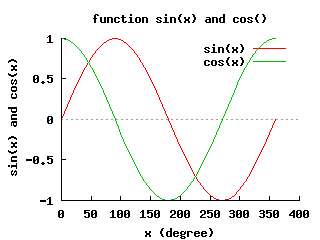/* The source code for generating the above plot */ /* File: legend.cpp Plot multiple sets of data in arrays using member function of plotting class CPlot */ #include #include #define N 37 /* number of points for the plot */ int main() { int i, num=0; double x[N], y[N], y2[N]; /* arrays with data for plotting */ class CPlot plot; /* instantiate a plotting class */ /* fill arrays with plotting data */ for(i=0; i